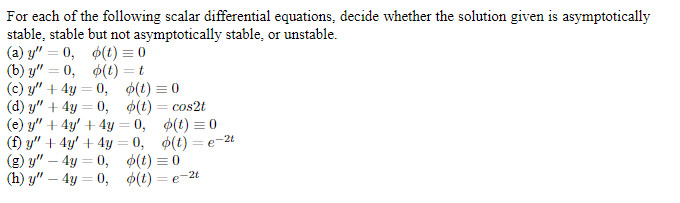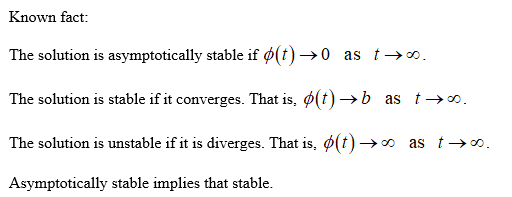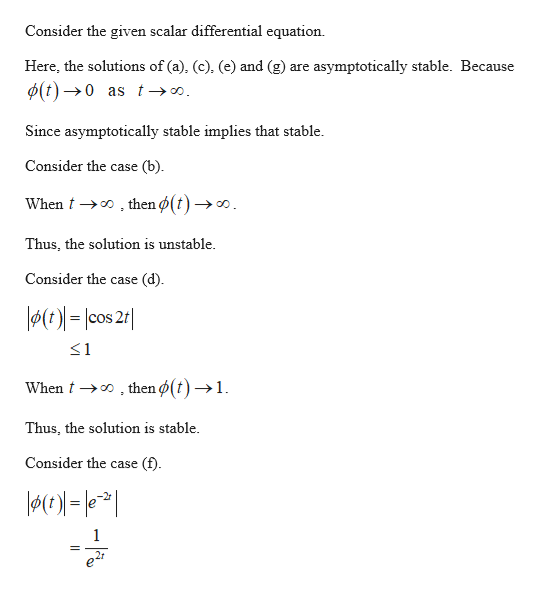# For each of the following scalar differential equations, decide whether the solution given is asymptoticallystable, stable but not asymptotically stable, or unstable(a) y"0, (t) 0(b) у" — 0, Ф(t) — t(c) y"4y 0, (t)0(d) y"4y 0, (t) = cos2t(e) y"4y4y 0, ¢(t)0(f) y"4y4y 0,

Question
44 viewshelp_outlineImage TranscriptioncloseFor each of the following scalar differential equations, decide whether the solution given is asymptotically stable, stable but not asymptotically stable, or unstable (a) y"0, (t) 0 (b) у" — 0, Ф(t) — t (c) y"4y 0, (t)0 (d) y"4y 0, (t) = cos2t (e) y"4y4y 0, ¢(t)0 (f) y"4y4y 0, <(t) = e-2t (g) y" 4y0, ¢(t) 0 (h) y" — 4у — 0, Ф(() — е- 2 fullscreen
check_circle

Step 1...help_outlineImage TranscriptioncloseConsider the given scalar differential equation Here, the solutions of (a), (c), (e) and (g) are asymptotically stable. Because d(t)0 as t-»o Since asymptotically stable implies that stable Consider the case (b) then ø(t) -»o. When Thus, the solution is unstable. Consider the case (d) )=lcos 2r <1 then ø(t) -»1 When Thus, the solution is stable Consider the case (f) 1 = fullscreen

### Want to see the full answer?

See Solution

#### Want to see this answer and more?

Solutions are written by subject experts who are available 24/7. Questions are typically answered within 1 hour.*

See Solution
*Response times may vary by subject and question.
Tagged in